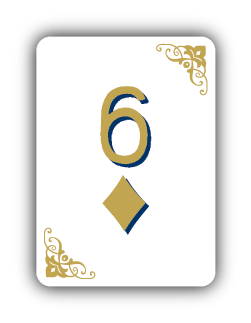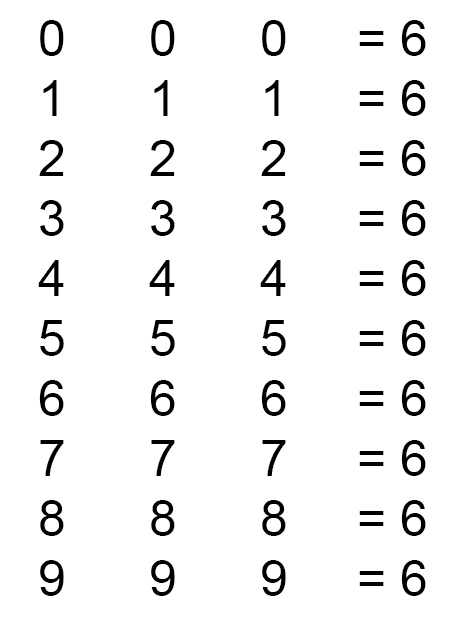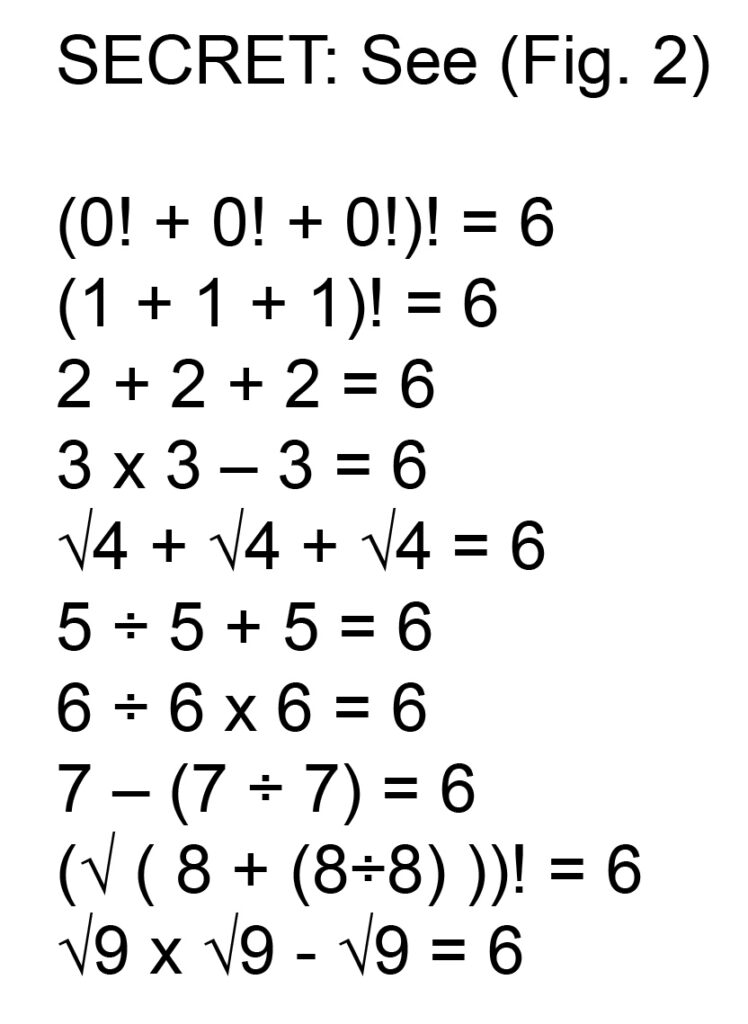## A number teaser to stump even the mathematicians.

Brain Teaser:

Write down each of the digits (0 through 9) three times side by side, then an equal sign, and then the number six (Fig. 1). Ask someone to make each of these equations true using only mathematical symbols. Insist that no extra numbers or digits of any kind can be added to any of the solutions. Further explain that none of the numbers values may be changed.

This is not easy. If you leave this mathematical riddle with a group they’ll typically work out the answers for half of them in about five to twenty minutes. It’s the other half that takes some doing.Solution:

The simple reason for this is that the common Joe doesn’t deal with square roots and factorials on a daily basis. Also, unless one makes a living with math or just finished taking a math class, they will not know that the definition of zero-factorial is “one”. So if you really want to stump someone then this is a great puzzle.

When presenting this on paper be sure to leave plenty of room for folks to add the necessary mathematical symbols (Fig. 1). You’ll find that many of the rows of numbers have multiple solutions for equaling six.

Showing folks math puzzles and solutions like this will make them think you are a genius. Once you’ve blown their mind with the mathematical mystery above follow it up by saying, “Did you also know that 111,111,111 x 111,111,111 = 12,345,678,987,654,321?” That’s 12 quadrillion, 345 trillion, 678 billion, 987 million, 654 thousand and 321.

Then ask, “Do you know the difference between being a genius and being stupid?” After hearing their insightful response tell them, “Genius has it limits, whereas stupidity knows no bounds.”For more commercial magic and puzzles from Diamond Jim Tyler visit his online Magic Shop!